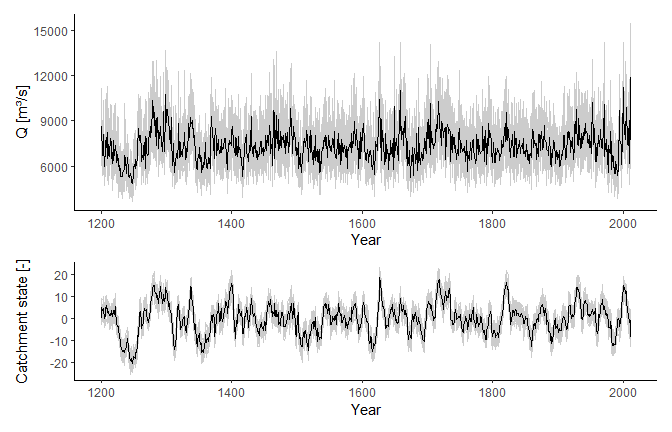# Streamflow Reconstruction with Linear Dynamical Systems

## Introduction

This package implements the Linear Dynamical System Expectation Maximization (LDS-EM) algorithm presented in Nguyen and Galelli (2018) to reconstruct streamflow (and possibily other climate variables) from paleoclimate proxies. The streamflow-proxy relationship is modeled as a linear dynamical system (LDS), following the set of equations

\begin{align} x_{t+1} &= Ax_t + Bu_t + q_t\\ y_t &= Cx_t + Dv_t + r_t\\ q_t &\sim \mathcal{N}(0,Q)\\ r_t &\sim \mathcal{N}(0,R)\\ x_1 &\sim \mathcal{N}(\mu_1, V_1) \end{align} where $$x_t$$ is the system state (the flow regime of the catchment), $$y_t$$ the (log-transformed) centralized streamflow, $$u_t$$ and $$v_t$$ the exogenous inputs, and $$q_t$$ and $$r_t$$ white noises. The system parameters are $$\theta = (A, B, C, D, Q, R, \mu_1, V_1)$$. Often, $$u$$ and $$v$$ are taken to be the same. For detail, please refer to Nguyen and Galelli (2018).

This package is the key workshorse behind Nguyen and Galelli (2018) and Nguyen et al (in prep). ldsr stands for Linear Dynamical System Reconstruction.

We will demonstrate the package using a part of Nguyen et al (in prep). Here, we reconstruct streamflow for the station Nakhon Phanom located along the Mekong River. The climate proxy is the portion of the Monsoon Asia Drought Atlas (MADA) (Cook et al, 2010) version 2 (Cook et al, 2015). The necessary data are already included in the package:

• NPannual is a data frame recording annual streamflow measured at station P1, which was obtained from the Thai Royal Irrigation Department. This record spans the period 1960–2005.
• NPpc is the three principal components (PCs 1, 9, and 13) selected from the MADA region surrounding Nakhon Phanom, following the procedure described in Nguyen et al (in prep). This record spans the period 1200–2012.

Some preparations:

We load the packages that are used frequently in this vignette. Other packages will be referred to with :: when necessary.

library(ldsr)       # This package
library(data.table) # Data wrangling
library(ggplot2)    # Plotting
library(patchwork)  # Arranging multiple plots

Preview data

head(NPannual)
#>    year       Qa
#> 1: 1960 7271.142
#> 2: 1961 8173.616
#> 3: 1962 6576.356
#> 4: 1963 8113.863
#> 5: 1964 8006.967
#> 6: 1965 7730.301
NPpc
#>             PC1        PC9        PC13
#>   1:  -5.748728 -1.0309588  1.48998471
#>   2:  -2.714017 -2.1571680 -1.12174381
#>   3:   4.824288 -1.1022443  1.12793587
#>   4:  -4.660712  0.4290133  0.03325184
#>   5:   3.141989  1.3189562 -1.16278505
#>  ---
#> 809:  -5.335305 -0.3152028 -0.90179438
#> 810:  -2.244408 -0.4942482 -2.20990129
#> 811:   3.601317  2.0233866  1.29098807
#> 812: -11.417119 -1.1643611 -6.54509700
#> 813:  -2.066818 -1.9109869 -1.35111091

## Reconstruction

Since EM is a local search routine, we run it with multiple restarts, each of which has a different initial condition. From our experience, about 20-50 restarts is sufficient. Computations can be sped up using parallel computing, and users can setup any parallel backend according to their system. We recommend the doFuture backend. On a 3.4 GHz quad-core desktop, the training procedure takes about a second with 20 restarts.

# Setup doFuture as the parallel computing backend
doFuture::registerDoFuture()
future::plan(future::multiprocess)
# Learn LDS
u <- v <- t(NPpc)
lds <- LDS_reconstruction(NPannual, u, v, start.year = 1200, num.restarts = 20)

### Model parameter

lds$theta #>$A
#>           [,1]
#> [1,] 0.8040856
#>
#> $B #> PC1 PC9 PC13 #> [1,] -0.3222316 -0.4333582 1.796134 #> #>$C
#>             [,1]
#> [1,] 0.008563054
#>
#> $D #> PC1 PC9 PC13 #> [1,] -0.01772445 -0.01587842 -0.04262785 #> #>$Q
#>         [,1]
#> [1,] 1.32991
#>
#> $R #> [,1] #> [1,] 0.01098105 #> #>$mu1
#>      [,1]
#> [1,]    0
#>
#> $V1 #> [,1] #> [1,] 1 ### Reconstruction result in the instrumental period p1 <- ggplot(lds$rec[year %in% NPannual$year]) + geom_ribbon(aes(year, ymin = Ql, ymax = Qu), fill = 'gray90') + geom_line(aes(year, Q, colour = 'LDS')) + geom_line(aes(year, Qa, colour = 'Observation'), data = NPannual) + scale_colour_manual(name = NULL, values = c('black', 'darkorange')) + labs(x = NULL, y = 'Mean annual flow [m\u00b3/s]') + theme_classic() + theme(axis.ticks.x = element_blank(), axis.line.x = element_blank(), axis.text.x = element_blank()) p2 <- ggplot(lds$rec[year %in% NPannual$year]) + geom_ribbon(aes(year, ymin = Xl, ymax = Xu), fill = 'gray90') + geom_line(aes(year, X)) + geom_hline(yintercept = 0) + theme_classic() + labs(x = 'Year', y = 'Catchment state [-]') p1 / p2 + plot_layout(heights = c(1, 0.6))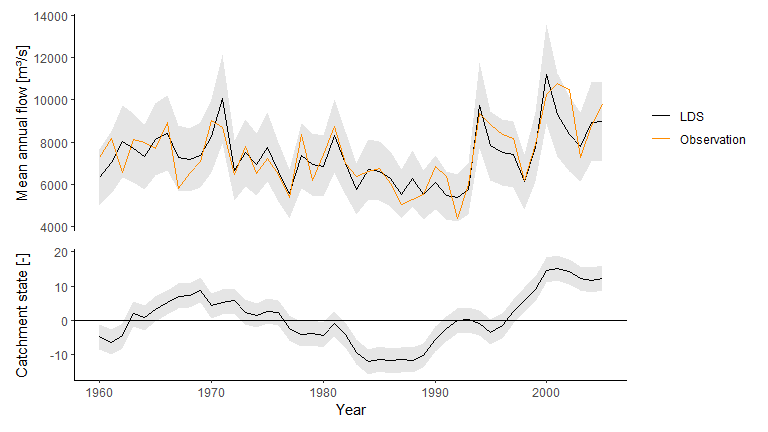The river has gone through distinct wet and dry epochs. ### Full time series p1 <- ggplot(lds$rec) +
geom_ribbon(aes(year, ymin = Ql, ymax = Qu), fill = 'gray90') +
geom_hline(aes(yintercept = mean(Q)), colour = 'salmon') +
geom_line(aes(year, Q)) +
labs(x = NULL, y = 'Mean annual flow [m\u00b3/s]') +
theme_classic() +
theme(axis.ticks.x = element_blank(),
axis.line.x = element_blank(),
axis.text.x = element_blank())

p2 <- ggplot(lds$rec) + geom_ribbon(aes(year, ymin = Xl, ymax = Xu), fill = 'gray90') + geom_hline(yintercept = 0, colour = 'salmon') + geom_line(aes(year, X)) + theme_classic() + labs(x = 'Year', y = 'Catchment state [-]') p1 / p2 + plot_layout(heights = c(1, 0.6))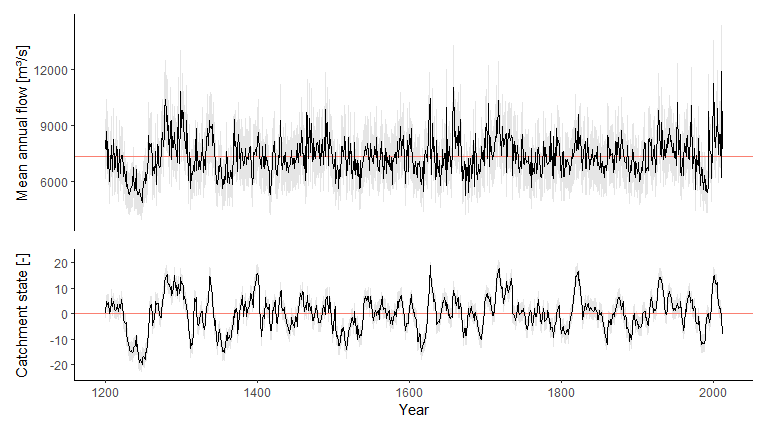## Cross-validation Make a set of cross-validation folds. set.seed(100) Z <- make_Z(NPannual$Qa, nRuns = 30, frac = 0.25, contiguous = TRUE)

Run cross-validation

cv <- cvLDS(NPannual, u, v, start.year = 1600, num.restarts = 20, Z = Z)

Cross-validation scores

cv$metrics #> R2 RE CE nRMSE KGE #> 1: 0.7403137 0.5747306 0.4304108 0.113233 0.6771942 ## Compare with linear regression Since LDS is a new method, which has not been through the test of time, we encourage users to thoroughly check the results, including comparing it against linear regressin. The package has some functions to do reconstruct streamflow with principal component linear regression (PCR). # Build PCR model pcr <- PCR_reconstruction(NPannual, NPpc, start.year = 1200) # Cross validate with the same folds as before cvpcr <- cvPCR(NPannual, NPpc, start.year = 1200, Z = Z, metric.space = 'original') ### Compare performance scores Mean performance scores rbind(lds = cv$metrics, pcr = cvpcr$metrics) #> R2 RE CE nRMSE KGE #> 1: 0.7403137 0.5747306 0.4304108 0.1132330 0.6771942 #> 2: 0.5813514 0.5038711 0.2909929 0.1282957 0.7073263 Performance scores over all cross-validation runs dt1 <- as.data.table(cvpcr$metrics.dist)
dt1[, model := 'PCR']
dt2 <- as.data.table(cv$metrics.dist) dt2[, model := 'LDS'] dt <- rbind(dt1, dt2) dt <- melt(dt, id.vars = 'model', variable.name = 'metric') ggplot(dt, aes(model, value)) + geom_boxplot() + stat_summary(geom = 'point', fun = mean, colour = 'red') + facet_wrap(vars(metric), scales = 'free', nrow = 1) + labs(x = NULL, y = NULL) + theme_classic() + theme(strip.background = element_rect(fill = 'gray90', colour = NA))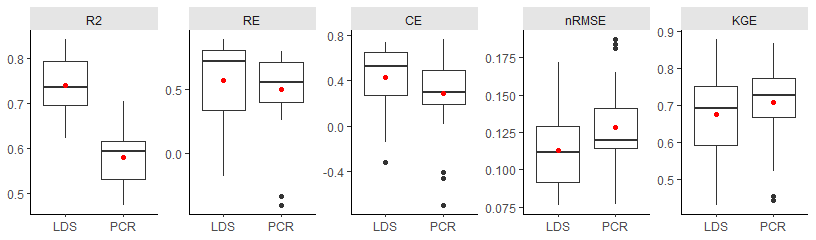### Compare reconstructions #### Instrumental period p1 <- ggplot(lds$rec[year %in% NPannual$year]) + geom_ribbon(aes(year, ymin = Ql, ymax = Qu), fill = 'gray90') + geom_line(aes(year, Q, colour = 'LDS', linetype = 'LDS')) + geom_line(aes(year, Q, colour = 'PCR', linetype = 'PCR'), data = pcr$rec[year %in% NPannual$year]) + geom_line(aes(year, Qa, colour = 'Observation', linetype = 'Observation'), data = NPannual) + scale_colour_manual(name = NULL, values = c('black', 'darkorange', 'black')) + scale_linetype_manual(name = NULL, values = c(1, 1, 2)) + labs(x = NULL, y = 'Mean annual flow [m\u00b3/s]') + theme_classic() + theme(axis.ticks.x = element_blank(), axis.line.x = element_blank(), axis.text.x = element_blank()) p2 <- ggplot(lds$rec[year %in% NPannual$year]) + geom_ribbon(aes(year, ymin = Xl, ymax = Xu), fill = 'gray90') + geom_line(aes(year, X)) + geom_hline(yintercept = 0) + theme_classic() + labs(x = 'Year', y = 'Catchment state [-]') p1 / p2 + plot_layout(heights = c(1, 0.6))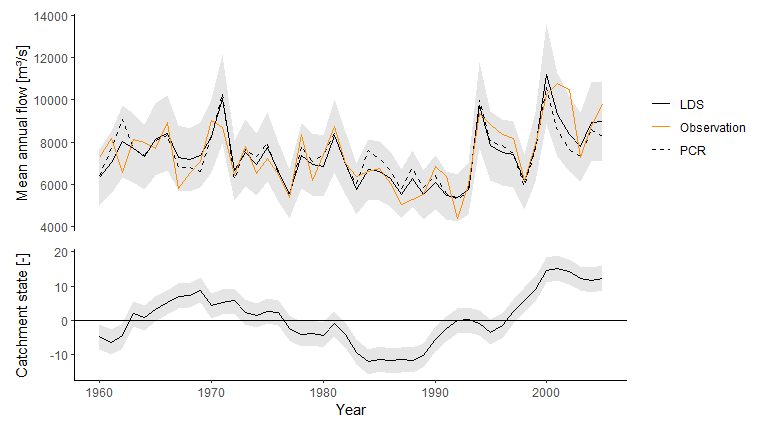#### Full horizon p1 <- ggplot(lds$rec) +
geom_ribbon(aes(year, ymin = Ql, ymax = Qu), fill = 'gray90') +
geom_line(aes(year, Q, colour = 'LDS', linetype = 'LDS')) +
geom_line(aes(year, Q, colour = 'PCR', linetype = 'PCR'), data = pcr$rec) + scale_colour_manual(name = NULL, values = c('black', 'steelblue')) + scale_linetype_manual(name = NULL, values = c(1, 2)) + labs(x = NULL, y = 'Mean annual flow [m\u00b3/s]') + theme_classic() + theme(axis.ticks.x = element_blank(), axis.line.x = element_blank(), axis.text.x = element_blank()) p2 <- ggplot(lds$rec) +
geom_ribbon(aes(year, ymin = Xl, ymax = Xu), fill = 'gray90') +
geom_line(aes(year, X)) +
geom_hline(yintercept = 0) +
theme_classic() +
labs(x = 'Year', y = 'Catchment state [-]')

p1 / p2 + plot_layout(heights = c(1, 0.6))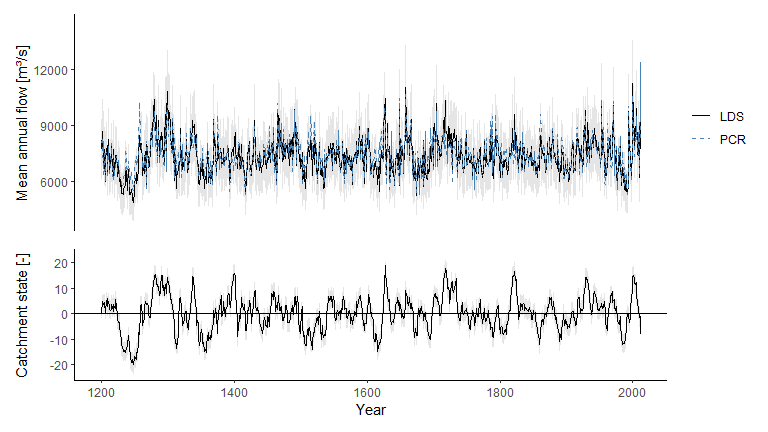## Stochastic replicates

An advantage of the LDS model is that it can be used readiliy as a stochastic streamflow generator.

Generate stochastic replicates

set.seed(100)
reps <- LDS_rep(lds$theta, u, v, years = lds$rec$year, mu = mean(log(NPannual$Qa)))

Plot the replicates

# Plot streamflow
p <- ggplot(reps) +
geom_line(aes(year, simQ, group = rep), colour = 'gray80') +
geom_line(aes(year, Q), data = lds$rec, colour = 'black') + labs(x = 'Year', y = 'Q [m\u00b3/s]') + theme_classic() # Plot catchment state q <- ggplot(reps) + geom_line(aes(year, simX, group = rep), colour = 'gray80') + geom_line(aes(year, X), data = lds$rec, colour = 'black') +
labs(x = 'Year',
y = 'Catchment state [-]') +
theme_classic()

p / q + plot_layout(heights = c(1, 0.6))